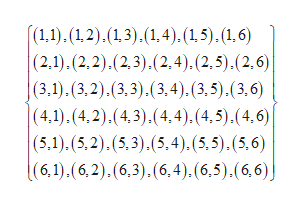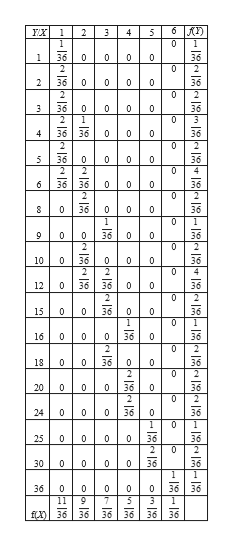# Two fair dice are rolled. Let X denote the smallest value of the two dice, and Y denote the product of the two dice.(a) Find fX,Y(x,y), fX(x), and fY(y) [you do not need to determine the mathematical function for these, it is fine to just list the probabilities].(b) Find prob(X=2|Y=12) and prob(Y=12|X=2).(c) Find E(Y|X=2). (d) Find Cov(X,Y).(e) Find Corr(X,Y).

Question
302 views

Two fair dice are rolled. Let X denote the smallest value of the two dice, and Y denote the product of the two dice.

(a) Find fX,Y(x,y), fX(x), and fY(y) [you do not need to determine the mathematical function for these, it is fine to just list the probabilities].

(b) Find prob(X=2|Y=12) and prob(Y=12|X=2).

(c) Find E(Y|X=2). (d) Find Cov(X,Y).

(e) Find Corr(X,Y).

check_circle

Step 1

Hello! As you have posted 5 sub parts, we are answering the first 3 sub-parts.  In case you require the unanswered parts also, kindly re-post that parts separately.

Step 2

(a).

From the given information, two fair dice are rolled.help_outlineImage Transcriptionclose(1.,1). (1,2).(13).(1,4). (1,5). (1,6) (2,1).(2,2).(2,3).(2,4). (2,5).(2,6) |(3,1).(3,2).(3,3),(3,4). (3,5) . (3, 6) (4,1).(4,2).(4,3).(4,4). (4,5) . (4, 6)| (5.1).(5,2).(5,3).(5.4). (5.5).(5,6) |(6,1).(6,2).(6,3).(6,4). (6,5) ,(6, 6)j fullscreen
Step 3

Let us define the random variable X as smallest value of the two...help_outlineImage Transcriptioncloseух 1234 0 1 0 0 0 0 3 0 0 0 4 0 0 0 0 0 0 9 0 0 0 10 0 0 0 12 0 0 15 16 18 0 0 0 0 0 20 0 0 0 00 24 00 0 25 0 30 36 f) O Oo fullscreen

### Want to see the full answer?

See Solution

#### Want to see this answer and more?

Solutions are written by subject experts who are available 24/7. Questions are typically answered within 1 hour.*

See Solution
*Response times may vary by subject and question.
Tagged in

### Probability